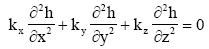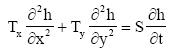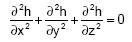# SAMPLE

 Page 1 [Page 2] Page 3 Page 4 Page 5 Page 6

Multiple Choice

(1 pt each)

2. (transient) Which of the following accurately describes the relationship between stress periods
and time steps in MODFLOW?

 a. Time steps are subdivided into stress periods. Boundary condition values can only change at the beginning of time steps. b. Time steps are subdivided into stress periods. Boundary condition values can only change at the beginning of stress periods. c. Stress periods are subdivided into time steps. Boundary condition values can only change at the beginning of time steps. d. Stress periods are subdivided into time steps. Boundary condition values can only change at the beginning of stress periods.

3. (modflow) Which of the following is not an accurate description of how evapotranspiration is
simulated in MODFLOW:

 a. Below a certain extinction depth, evapotranspiration is zero. b. Above a certain elevation, evapotranspiration occurs at a maximum rate. c. Between the two elevations in a and b, evapotranspiration varies linearly between zero and the maximum rate. d. Evapotranspiration is always a sink, not a source. e. All of the above are true statements.

4. (calibration) Which of the following is NOT used as an observation when calibrating a MODFLOW model?

 a. Measured recharge flux resulting from rainfall. b. Measured gains and losses along head dependent boundary conditions. c. Heads at observation wells. d. All of the above can be used as observations.

5. (transient) For a 2D unconfined groundwater model, or an unconfined layer in MODFLOW, the storage term is called:

 a. Specific storage b. Storativity c. Specific yield

6. (transient) For a steady state model, which of the following statements are true about the starting head array?

 a. It doesn't matter what values are used because MODFLOW will converge to the same final result. b. The values should be found from a previous simulation. Otherwise, in the early time steps the model will react to the ill-posed boundary conditions rather than to the real model stimuli. c. There may be many combinations of values that will give the same solution. However, you may still have problems if the heads are too low or if they differ too much from the final heads. d. None of the above.

 a. The change in total head divided by the gross cross sectional area of flow. b. The change in pressure head divided by the gross cross sectional area of flow c. The change in total head divided by the flow distance. d. The change in pressure head divided by the flow distance. e. None of the above.

8. (bc) Which of the following does not represent a no-flow boundary condition?

 a. A ground water divide. b. A large river c. A rock outcropping d. A parallel flow boundary e. All of the above are no-flow boundaries.

9. (calibration) Which of the following error norms is generally the largest?

 a. Mean error b. Mean absolute error c. Root mean squared error

10. (gw-concepts) Which of the following names is typically used to describe a model that is developed primarily to gain a better understanding of an aquifer system (flow rates, head distributions, flow budget, etc.)?

 a. Predictive b. Interpretive c. Analytical d. Generic

11. (bc) It is OK to use specified head boundaries corresponding to water table contours on the local model when doing regional to local model conversion because:

 a. The boundaries are a large distance from the area of interest. b. The model parameters (K and recharge) have already been calibrated using the regional model. c. The head contours are interpolated from observed heads at observation wells. d. It guarantees that you will get good model calibration.

12. (modpath) Which of the following best describes the function of the zone code used with MODPATH?

 a. It is used to "parameterize" the model input. b. It defines regions with different porosity c. It can be used to control how the pathlines are displayed. For example, you can color the pathlines according to the zone code of where the paths begin or terminate. d. It controls how many particles are placed per cell.

13. (transient) Which of the following represents the amount of water released from a unit area of a confined aquifer due to a unit decline in head?

 a. Specific storage b. Specific yield c. Storativity d. None of the above

14. (gms) The units for the value of conductance assigned to arcs in the GMS Map Module for stress packages (River, Drain, General Head, etc.) associated with MODFLOW is:

 a. [L]/[T^2] b. [L]/[T] c. [L^2]/[T] d. /[T] e. None of the above

15. (goveq) For the governing equation:which of the following conditions apply?

 a. isotropic b. anisotropic c. xyz axes aligned with principal axes of hyd. conductivity d. 2D e. 3D f. steady state

16. (goveq) For the governing equation:which of the following conditions apply?

 a. isotropic b. anisotropic c. 2D d. 3D e. steady state f. transient

17. (goveq) For the governing equation:which of the following conditions apply?

 a. isotropic b. anisotropic c. internal sources and sinks d. steady state e. 2D